MorphEngine: Custom Data TypesMorphEngine supports custom data types. These are “first-class.” That is, they can be as fully-featured as, and indistinguishable in look & feel from, built-in types. They can also “mix” and “mesh” with built-in types.

A custom type is typically defined as a Code object in a database folder and permanently “injected” into the calculator by the user. The Code object is commonly called “injection” and the injection commonly happens through a one-line RPL program, named “install”, that looks like this:

≪ "typename" injection inject ≫ (where typename is the name you choose for your type)

A custom data type can very easily use external sources for its definition. This includes any JS framework and external .js files. If .js files are provided, they are typically listed as URL assets in the folder that defines the type and downloaded by the user via Sharing | Download Assets.

In this section, we introduce the concept, discuss the custom data type framework, and look in detail at four custom data type examples: Code, ChemFormula, NDImage, BigNum.

The last of these, BigNum, is a fully-fleshed example that shows how a type that intimately interacts with built-in types (Binary and Real Numbers, Arrays), can be integrated with very little added code and without changing one line in external files (comprising two thousand lines of code, in this case) that define a calculation object, which have no knowledge of MorphEngine whatsoever.

This section can be read independently from other MorphEngine documentation and will enable you to write your own custom data types–simple and complex.

Overview

The wrapper structure looks as follows:

var MyType = {

type: "mytype",

isLoaded: false, // whether or not type is available; usually 'true' if nothing needs to be loaded, or set to 'true' in onload after required extensions are loaded

toString: function(obj) { return obj.toString(); },

toHTML: function(obj) { return (this.toString() + calculator.HTMLforTypeBadge(obj.type)); },

fromString: function(str) { return new MyObj(str); }, // optional; if present, string rep will be used for editing

isStringRepresentation: function(str) { return (str.match(/someRegExpOrOtherTest/) != null); }, // optional; used by push for unknown item to test membership of this type

if (!require("MyType.js"))

}

};

The wrapper “class” is the single instance of an object, containing the following mandatory properties:

toString() a function returning a string representing your type; this can be any full or abbreviated, raw or descriptive, user-facing representation

In addition to these, a number of optional properties are defined, which MorphEngine will use, if they’re present:

toHTML() a function returning a string in HTML format, to be used for display on the stack. If this function is not present, the toString() result will be used for stack display.

fromString() a function that takes a string and returns an instance of your worker object. If this function is present, your type will become editable on the edit line. This function is only applicable if your type can be fully represented as a string, and if it makes sense for users to see this string. (An image, for example, might be representable as a string, but it would hardly make sense to represent a million random-looking characters to a user.)

isStringRepresentation() a function that takes a string and should return true, if the string is recognized to be a valid representation of your type, and, false, otherwise.

onload() a function, taking and returning nothing, that will be called when the calculator is initializing. You can use this to do static initializations.

onlyOperatesOnOwnType a boolean property that, when set to true, ensures that MorphEngine will attempt to convert other types to our type before passing arguments to functions that take more than one value.

The Wrapper Class

A custom data type in MorphEngine has two parts:

1. -a data/worker object, which holds your type’s data and which can be instantiated

2. -a “static” wrapper class, which provides MorphEngine glue

It’s your choice if you want to separate these two parts in formal “classes” and, if you do so, in which class “computation” (if there is any) will happen. The design is meant to lend itself toward having a worker class that knows nothing about MorphEngine, and a small wrapper class that integrates it into MorphEngine.

Since the engine only interfaces with the wrapper class, only it has a defined structure.

Data Object and Wrapper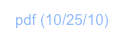Let’s dive right into the first example and illustrate the concepts above with concrete code.

var Code = {

1:     type: "code",

3:

4:     toString: function(obj) { return obj.stringValue; },

5:     toHTML: function(obj) {

6:         var stringForType = obj.stringValue.slice(0, display.isLarge() ? 60 : 15) + " (" + obj.stringValue.length + "b)";

7:         return (stringForType + calculator.HTMLforTypeBadge(obj.type, display.isLarge() ? 43 : 37));

8:     },

9:     isStringRepresentation: function(x) { return x.match(/^\/\*/); },

10:    fromString: function(str) {

11:        function codeObj() {

12:            this.type = Code.type;

13:            this.stringValue = str;

14:            this.toString = function() { return Code.toString(this); };

15:        }

16:        return new codeObj();

17:    },

18:

19:    "==": function(a, b) { if (!(calculator.typeOf(a) == "code" && calculator.typeOf(b) == "code")) return false; return a.stringValue == b.stringValue; },

20:    "inject": function(name, obj) { if (calculator.typeOf(name) != "string") throw Error("bad arg"); calculator.exec("inject", obj.stringValue, calculator.unquote(name)); },

21:

22:    eval: function(obj) { return eval(obj.stringValue); },

23:

25:        // add a global constant

26:        calculator.vars["noCode"] = Code.fromString("");

27:    }

};

A Code object is a container for JavaScript code.

Here’s an example of how a Code object displays on the stack: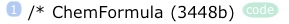Requirements for this data type are as follows:

1. -Let user enter arbitrary code on the edit line

2. -Let code objects reside on stack, where the user should just see the beginning of the code text, the number of bytes in the text, and a pretty badge reading “code”

3. -Let user evaluate the code to perform a syntax check

4. -Let user compare two code objects and see if they’re identical

5. -Let user inject code into the calculator

6. -Provide a global variable that represents an empty code object

These goals are accomplished with the object above, which defines both the wrapper and, in lines 11-15, the instantiable “worker” object.

Let’s look at each line of this object, following a logical flow:

Line 1 type is simply the desired name we want the data type to have

Line 2 isLoaded tells MorphEngine that the type is available and ready. Typical for a data type with no external dependencies, we just statically say “yes” here

Line 9 isStringRepresentation() is an optional function but it’s crucial for this type, because we want that the user can type in text data, that will be used to construct an instance of this type. In this function, we perform a simple test of the first two characters of a given candidate string. If the string begins with “/*”, we’ll want to claim this input and make it an instance of our type. MorphEngine will present us any input the user types, and offer us to make this claim. If this function weren’t present, or we were never to say “yes” here, the user would not be able to create an instance of our data type by typing text (appropriate for our type) on the edit line

Line 10 fromString() is what follows after MorphEngine hears back from us that we want to use the offered string to make an object

Lines 11 through 15 define the instantiable worker object that will hold our JavaScript source code. This object has just two properties (a.k.a. “members,” “instance variables”):

Line 12: type, our type name, taken right as a copy of our static type name from the wrapper object

Line 13: stringValue, a string holding our source code, which is assigned the literal string we’re given as input

We also define a “member” function:

Line 14: toString(), pointing to our wrapper’s static toString() function, invoked with ourselves (from the perspective of the worker object) as argument

In line 16 a new instance of our worker object is created and returned to MorphEngine

MorphEngine will hold our object on the stack, display it, and direct user commands to our wrapper class, if they match names of functions we define.

To display our object, MorphEngine first looks if we have a toHTML() function in our wrapper class. We do. It then passes it the instance in question. We can be sure that whenever this function is called, we’ll be given a valid worker class instance, with properties known to us–”stringValue” and “type” in our case.

Line 6 accesses the source code in “stringValue” and slices the first few characters off it. (It makes the numbers of characters dependent on a MorphEngine API call: display.isLarge(), which will return “yes”, if we’re on iPad, and “no”, if we’re on iPhone or iPod touch.) It also appends the count of characters in the source code in parentheses

Line 7 uses another MorphEngine API call, calculator.HTMLforTypeBadge()–which returns HTML code for a badge for a given string–appends it to our abbreviated and sized code bit, and returns it to MorphEngine for display

Now, we have three properties defined in our wrapper class that specify commands/functions that the user may exercise (directly by issuing a command; or indirectly, through programs) on objects of our type: “==”, “inject”, and “eval”. MorphEngine uses introspection of our wrapper class to discover which functions we define and will call them appropriately, without further involvement from us.

If the user taps the Eval key with a Code object at the first position on the stack, our function wrapper’s “eval” function, in line 22, will be called.

Line 22 simply calls eval() on our JavaScript source code (which we access through the known “stringValue” property). Any parse or syntax errors will be thrown and displayed to the user through MorphEngine catch code. (This line is the only simplified line in this example. The actual shipping version of the Code object in ND1 does a few more things here to correctly, and more prettily, report errors.)

The next two functions require us to do some type checking because we define two input parameters for these functions and MorphEngine will only guarantee that one of them is an instance of our type.

If the user types the command “inject”, or, more likely, if the “install” RPL program hits an “inject” instruction, and there’s a Code object at either the first or second position on the stack, our function “inject”, in line 20, will be called.

Line 20 first makes sure that the first parameter is a string, then calls the MorphEngine API function that injects a named piece of code into the calculator. (Such injections appear under JS Injections on the calculator’s Definition page.)

Similarly, if the user taps ‘==’ with two Code objects on the stack, our “==” function will be called.

In Line 19, we first check the type of the inputs using the calculator.typeOf() API function, which unsurprisingly returns our own type string for objects of our type, and then do a string comparison of the two source code texts, and return the result

Now you will fully understand the install program quoted in this section’s overview:

"mytype" injection inject ≫

Given a Code object–that a user could construct by entering text like this

/* My Type */

var MyType = {

type: "mytype",

...

};

and storing it under a variable named “injection”–this RPL code will invoke Code’s “inject” function with a type name and the given source code text. (The type name merely serves as a handle to find the code again, and permit reinstalls and uninstalls.)

If the MyType object shown had useful text in the “...” part, there’d just be one little thing missing to actually “activate” the type defined by this object:

calculator.registerType(MyType);

registerType() is the special API call that–when run at calculator initialization time, as part of over user injection processing–makes a type known to MorphEngine and makes it “go live.”

In summary, the complete code for injection of a custom data type–the “payload” of a Code object–looks like this:

/* My Type */

var MyType = {

type: "mytype",

...

};

calculator.registerType(MyType);

Example: Code

ChemFormula is a data type with the following user requirements:

1. -Let the user enter chemical formulas

2. -Have them appear nicely formatted on the stack

3. -Let the user eval them and return the formula’s relative molar mass as a result

This is an example of how a ChemFormula object displays on the stack: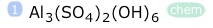Here’s the full code to accomplish these tasks:

var ChemFormula = {

type: "chem",

toString: function(obj) { return obj.stringValue; },

toHTML: function(obj) {

var stringForType = this.toString(obj).replace(/[\*\[\]] /g, "").replace(/[1-9]+(?!\ )/g, "<sub>\$&</sub>");

},

isStringRepresentation: function(x) { return x.match(/[A-Z][a-z]?([1-9]+|([A-Z][a-z]?)|[\(\)])+/); },

fromString: function(str) {

function formulaObj() {

this.type = ChemFormula.type;

this.stringValue = str;

this.toString = function() { return ChemFormula.toString(this); };

}

return new formulaObj();

},

"Mr": function(obj) { // relative molar mass

var atomicWeights = { // relative atomic mass, IUPAC 2007 data

"H": 1.00794, "He": 4.002602, "Li": 6.941, "Be": 9.012182, "B": 10.811, "C": 12.0107, "N": 14.0067, "O": 15.9994,

"F": 18.9984032, "Ne": 20.1797, "Na": 22.98976928, "Mg": 24.305, "Al": 26.9815386, "Si": 28.0855, "P": 30.973762, "S": 32.065,

"Cl": 35.453, "Ar": 39.948, "K": 39.0983, "Ca": 40.078, "Sc": 44.955912, "Ti": 47.867, "V": 50.9415, "Cr": 51.9961,

"Mn": 54.938045, "Fe": 55.845, "Co": 58.933195, "Ni": 58.6934, "Cu": 63.546, "Zn": 65.38, "Ga": 69.723, "Ge": 72.64,

"As": 74.9216, "Se": 78.96, "Br": 79.904, "Kr": 83.798, "Rb": 85.4678, "Sr": 87.62, "Y": 88.90585, "Zr": 91.224,

"Nb": 92.90638, "Mo": 95.96, "Tc": 98, "Ru": 101.07, "Rh": 102.9055, "Pd": 106.42, "Ag": 107.8682, "Cd": 112.411,

"In": 114.818, "Sn": 118.71, "Sb": 121.76, "Te": 127.6, "I": 126.90447, "Xe": 131.293, "Cs": 132.9054519, "Ba": 137.327,

"La": 138.9054519, "Ce": 140.116, "Pr": 140.90765, "Nd": 144.242, "Pm": 145, "Sm": 150.36, "Eu": 151.964, "Gd": 157.25,

"Tb": 158.92535, "Dy": 162.5, "Ho": 164.93032, "Er": 167.259, "Tm": 168.93421, "Yb": 173.054, "Lu": 174.9668, "Hf": 178.49,

"Ta": 180.94788, "W": 183.84, "Re": 186.207, "Os": 190.23, "Ir": 192.217, "Pt": 195.084, "Au": 196.966569, "Hg": 200.59,

"Tl": 204.3833, "Pb": 207.2, "Bi": 208.9804, "Po": 209, "At": 210, "Rn": 222, "Fr": 223, "Ra": 226, "Ac": 227,

"Th": 232.03806, "Pa": 231.03588, "U": 238.02891, "Np": 237, "Pu": 244, "Am": 243, "Cm": 247, "Bk": 247, "Cf": 251,

"Es": 252, "Fm": 257, "Md": 258, "No": 259, "Lr": 262, "Rf": 265, "Db": 268, "Sg": 271, "Bh": 272, "Hs": 270,

"Mt": 276, "Ds": 281, "Rg": 280, "Cn": 285, "Uut": 284, "Uuq": 289, "Uup": 288, "Uuh": 293, "Uuo": 294

};

calculator.vars.local = atomicWeights; // make atomicWeight our new set of local vars

var atomicWeight = calculator.eval(obj.stringValue.replace(/\[/g, "(").replace(/\]/g, ")").replace(/([A-Z][a-z]?)([0-9]+)/g, "\$1*\$2").replace(/([A-Z][a-z]?)|(\()/g, "+\$&"));

calculator.vars.local = {}; // reset local vars

return atomicWeight;

},

eval: function(obj) { return ChemFormula.Mr(obj); }

};

calculator.registerType(ChemFormula);

Our isStringRepresentation() function is a bit more complicated regular expression this time around, which checks if the candidate string is a sequence of one or two letters followed by numbers and possibly containing parentheses. If so, we identify the input string as chemical formula and claim it as “ours.”

In fromString() we create an instantiable worker object that’s almost identical to Code’s: we have a stringValue, assumed to be the string provided by the user, and a type property, which now maps to our new type’s name “chem”.

When the user taps Eval, we call our wrapper’s “Mr” function, which extracts the formula text from our object, and computes the relative molar mass using a bit of clever code that extracts coefficients, looks up atomic mass values for extracted element names, builds up a mathematical expression that can be evaluated, and then uses an API function, calculator.eval(), to evaluate the expression and return the relative molar mass value.

(We could have also use the standard eval() function to evaluate the expression, by the way. We use the calculator’s, because it will also allow for variables in our expression.)

The actual, shipping version of ChemFormula looks like this, but also adds a couple of operators, “+” and “*”, to permit our chemical formulas to be added and multiplied by scalars. You can see the full source code by downloading the Public data for this type, folder “Chem”.

Example 2: ChemFormula

NDImage is a type that represents a binary or gray or color image.

Constructed from binary data, it is meant to be a container type with only one user function, eval (or “toDisplay”), to display the image.

stringValue does not play a role in this type’s representation. The type does not support a fromString() function, and it cannot be edited on the edit line. It does, however, have a toString() function to provide a textual (descriptive) representation for display on the stack. But, unlike in the other examples, that string cannot be used to recreate the type.

Here’s how an image might display on the stack: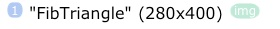Here’s the full injection code:

var NDImage = {

type: "img",

toString: function(obj) { return obj.name + " ("+ obj.width + "x" + obj.height + ")"; },

toImage: function(name, data, w, h) {

if (!(calculator.isAFirmString(name) && calculator.isAFirmString(data) && typeof w === 'number' && typeof h === 'number'))

throw Error("wrong type of argument");

data = data.slice(1,-1); // remove firm string quotes

if (!calculator.isAHexNumber(data))

throw Error("hexDataExpected");

data = data.slice(2); // shave off "0x"

var wantsBinaryInterpretation = false;

if (data.length == w*h*2) // required data size for gray image

;

else if (!(w&7) && data.length == w/8*h*2) // required data size for binary image

wantsBinaryInterpretation = true;

else

throw Error("invalidDimensionsForData");

}

// with inputs verified, it's time to construct the stack object

function imageObj() {

this.type = NDImage.type;

this.name = name;

this.width = w;

this.height = h;

this.data = data;

this.isBinary = wantsBinaryInterpretation;

}

return new imageObj();

},

toHTML: function(obj) {

return '<img src=' + obj.data + ' width="184" height="50" ' + '/>';

else {

var typeString = this.type;

var stringForType = this.toString(obj);

return (stringForType + calculator.HTMLforTypeBadge(typeString, display.isLarge() ? 37 : 30));

}

},

toDisplay: function(obj) {

display.showImage(obj);

else {

display.showGraphics(true);

var ctx = canvas.getContext('2d');

var image = ctx.createImageData(obj.width, obj.height);

var imageData = image.data;

var index = 0;

for (var i=0; i<imageData.length; index+=2) {

var val = parseInt(obj.data.substring(index,index+2), 16);

if (obj.isBinary) {

for (var s=7; s>=0; s--, i+=4) {

var bitVal = val&(1<<s);

imageData[i] = imageData[i+1] = imageData[i+2] = (bitVal ? 255 : 0);

imageData[i+3] = 255; // alpha set to "opaque"

}

}

else { // gray interpretation

imageData[i] = imageData[i+1] = imageData[i+2] = val;

imageData[i+3] = 255; // alpha set to "opaque"

i += 4;

}

}

ctx.putImageData(image, 0, 0);

}

},

eval: function(obj) { this.toDisplay(obj); },

// extend built-in functions

calculator.functions.string["toImage"] = NDImage.toImage;

// define function name aliases

calculator.function_aliases["image"] = "toImage";

}

};

calculator.registerType(NDImage);

Note, the “constructor” is the toImage() function which takes four arguments: a name for the image, a hexadecimal string containing the binary data, and two integers for dimensions.

This function can be called from a program, or input by the user (with the arguments expected by it on the stack), to successfully create an image. Such an image can then be displayed by issuing a “toDisplay” command, or tapping Eval (which just calls through to toDisplay).

Example 3: NDImage

BigInt is a data type that adds arbitrary precision integer support to ND1.

Based on Tom Wu’s excellent BigInteger class, which he made available in two JavaScript implementation files, jsbn.js and jsbn2.js, there was a goal of not changing any of his code.

ND1 offers downloads of assets, and MorphEngine allows sourcing of JavaScript files, via its ‘require()’ API call.

A type wrapper was created that loads the original BigInteger implementation files, and then wraps many of its functions under wrapper names that are consistent with ND1 keys and function naming of the similar Binary and Real Number types.

The integration also “mixes”–allowing operations of mixed types, Binary/Real and BigInt–and “meshes”, where built-in internal functions “!” (factorial) and “fib” (Fibonacci numbers) where changed so that for certain input value ranges the previous built-in function is deployed, and for others, the extended BigInt version.

All the while, the object on the calculator’s stack–its internal representation–is the original (!) BigInteger object, which only had its prototype expanded by one property: “type”, which is, just like in the examples above, the wrapper’s static type string.

Here’s an example of how a BigInt displays on the stack: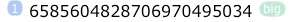Excluding straightforward lines that do simple mappings like this

"+": function(a, b) { return a.add(b); },

"-": function(a, b) { return a.subtract(b); },

the entire intimate integration is achieved with less than a hundred lines of code. (A basic integration is achieved with approx. 20 lines of code.)

Here’s the full injection code, with no simplifications applied:

var BigNum = {

type: "bignum",

onlyOperatesOnOwnType: true,

toString: function(bn) {

return (BigNum.radix == 16 ? (str == "-" ? "-0x" + str.slice(1) : "0x" + str) : (BigNum.radix == 2 ? str + "b" : (BigNum.radix == 8 ? str + "o" : str)));

},

toHTML: function(bn) {

var typeString = "big"; // user-visible type name; could also be this.type

var stringForType = BigNum.toString(bn);

return (stringForType + calculator.HTMLforTypeBadge(typeString, display.isLarge() ? 33 : 27));

},

fromBigNum: function(bn) { return this.toString(bn); },

fromAny: function(x) { return (typeof x === 'number' ? BigNum.fromNumber(x) : BigNum.fromString(String(x))); },

fromNumber: function(x) { x = Math.round(x); return BigNum.fromString(String(x)); },

fromString: function(str) { var base = 10;

if (BigNum.isStringRepresentation(str)) {

if (calculator.isAHexNumber(str)) {

str = str.slice(2); // shave off "0x"

base = 16;

}

else if (calculator.isATrueBinaryNumber(str)) {

str = str.slice(0, -1); // shave off trailing "b"

base = 2;

}

else if (calculator.isAnOctNumber(str)) {

str = str.slice(0, -1); // shave off trailing "o"

base = 8;

}

// default is dec / base 10

}

else if (calculator.isABinaryNumber(str))

str = String(calculator.functions.binary.toDec(str));

return new BigInteger(str, base);

},

isStringRepresentation: function(x) { return calculator.isABinaryNumber(x) && (x.length > (calculator.isATrueBinaryNumber(x) ? 31 : 14)); },

eval: function(obj) { return ("stringValue" in bn ? BigNum.fromString(obj.stringValue) : obj); },

"==": function(a, b) { return a.equals(b); },

"=": function(a, b) { return this["=="](a,b); },

"!=": function(a, b) { return !a.equals(b); },

">": function(a, b) { return a.compareTo(b) > 0; },

"<": function(a, b) { return a.compareTo(b) < 0; },

">=": function(a, b) { return a.compareTo(b) >= 0; },

"<=": function(a, b) { return a.compareTo(b) <= 0; },

"+": function(a, b) { return a.add(b); },

"-": function(a, b) { return a.subtract(b); },

"*": function(a, b) { return a.multiply(b); },

"/": function(a, b) { return a.divide(b); },

"toBin": function(a) { this.radix = 2; return a; },

"toOct": function(a) { this.radix = 8; return a; },

"toDec": function(a) { this.radix = 10; return a; },

"toHex": function(a) { this.radix = 16; return a; },

"neg": function(a) { return a.negate(); },

"sign": function(a) { return a.signum(); },

"mod": function(a, b) { return a.mod(b); },

"max": function(a, b) { return a.max(b); },

"min": function(a, b) { return a.min(b); },

"abs": function(a) { return a.abs(); },

"size": function(a) { return a.bitLength(); },

"gcd": function(a, b) { return a.gcd(b); },

"lcm": function(a, b) { return BigNum["*"](BigNum["/"](a, this.gcd(a,b)), b); },

"pow": function(a, b) { return a.pow(b); },

"^": function(a, b) { return this["pow"](a,b); },

"shift_left": function(a) { return a.shiftLeft(1); },

"shift_right": function(a) { return a.shiftRight(1); },

"shift_left_byte": function(a) { return a.shiftLeft(8); },

"shift_right_byte": function(a) { return a.shiftRight(8); },

"and": function(a, b) { return a.and(b); },

"or": function(a, b) { return a.or(b); },

"xor": function(a, b) { return a.xor(b); },

"not": function(a) { return a.not(); },

"incr": function(bn) { return BigNum["+"](bn, BigInteger.ONE); },

"decr": function(bn) { return BigNum["-"](bn, BigInteger.ONE); },

"factorial": function(bn) { return (BigNum["<="](bn, BigInteger.ONE) ? BigInteger.ONE : BigNum["*"](bn, arguments.callee(BigNum["-"](bn, BigInteger.ONE)))); },

"fib": function(bn) { if (BigNum["<"](bn, BigInteger.ZERO)) throw Error("invalid arg");

var a = BigInteger.ZERO; var one = BigInteger.ONE; var b = one;

var maxNum = parseInt(bn.toString());

for (var i=1; i<maxNum; i++) {

var val = BigNum["+"](a, b);

a = b; b = val;

}

return val;

},

if (!require("jsbn", true))

return;

if (!require("jsbn2"))

// extend prototype

BigInteger.prototype.type = BigNum.type;

BigInteger.prototype.toJSON = function(key) { return { "type": BigNum.type, "needsRevival": true, "stringValue": this.toString() }; };

// blend type's functions into calculator

calculator.functions["toBigNum"] = BigNum.fromAny;

// extend built-in functions

calculator.functions.binary["toBigNum"] = BigNum.fromString;

// use BigNum version for certain arg ranges to certain functions, use built-in otherwise

calculator.functions["fib_real"] = calculator.functions["fib"]; // move built-in function to new place

calculator.functions["fib"] = function(x) { return (x>77 ? BigNum.fib(BigNum.fromNumber(x)) : calculator.functions["fib_real"](x)); };

calculator.functions["factorial_real"] = calculator.functions["factorial"]; // move built-in function to new place

calculator.functions["factorial"] = function(x) { return (x>99 ? BigNum.factorial(BigNum.fromNumber(x)) : calculator.functions["factorial_real"].call(calculator.functions, x)); };

// define function name aliases

calculator.function_aliases["\u2192big"] = "toBigNum";

calculator.function_aliases["big\u2192"] = "fromBigNum";

}

};

calculator.registerType(BigNum);

Note the two require() statements in onload() : require() dynamically loads JavaScript source files, from either a remote (http://) location, or locally. Source files may appear as URL assets in an extension’s folder, and require download (via Sharing | Localize Assets) before they can be successfully referenced as local sources. (See the jsbn.js and jsbn2.js entries in the BigInt folder.)

Note the use of onlyOperatesOnOwnType. This property set to true ensures that MorphEngine will attempt to convert other-than-BigInt types to BigInt before passing args to functions that take more than one argument. This saves all type checking in this class, and has the effect of automatically “promoting” the related types–Binary and Real Number–to BigInt. The conversion attempt involves converting the type in question to a string (which is usually possible; if not an exception will be thrown) and creating a BigNum instance from this string, via BigNum’s fromString() function.

Note the implementation of the factorial function in BigInt:

"factorial": function(bn) { return (BigNum["<="](bn, BigInteger.ONE) ? BigInteger.ONE : BigNum["*"](bn, arguments.callee(BigNum["-"](bn, BigInteger.ONE)))); },

After injection, BigNum is a first-class type in the calculator. That also implies that a user, or developer, can go out and write code like this factorial function, which refers to, and uses, the type.

Each of the scenarios for extending MorphEngine (as described in MorphEngine Concepts) apply: a user can overwrite, extend, and add to the BigNum type, just like they can with a built-in type, like Real Number.

Example 4: BigInt